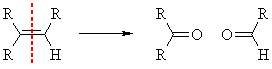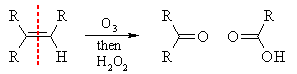# What are the products obtained after ozonolysis of the following compounds: 2,4,4-trimethylpent-2-ene; 2-methylbut-1-ene; 1-methylclohexene?

Jul 13, 2017

Ketones, and aldehydes, depending on workup......

#### Explanation:

${R}_{1} {R}_{2} C = C {R}_{3} {R}_{4} \stackrel{{O}_{3}}{\rightarrow} {R}_{1} {R}_{2} C = O + O = C {R}_{3} {R}_{4}$.

While aldehydes are unstable with respect to oxidation, you should be able to chop up an olefin to give a series of aldehydes and ketones. If you can individually identify each oxo-compound, you could evidence the structure of the original olefin by wet chemical methods.

Jul 13, 2017

The products depend on the nature of the workup.

Reducing workup

This involves reaction with ozone, then workup with $\text{Zn/acetic acid}$ or ("CH"_3)_2"S".

The process cleaves the alkene into aldehydes and/or ketones.(a) 2,4,4-Trimethylpent-2-ene

$\left(\text{CH"_3)_3"CH=C"("CH"_3)_2 → underbrace(("CH"_3)_3"CH=O")_color(red)("2,2-dimethylpropanal") + underbrace("O=C"("CH"_3)_2)_color(red)("propanone}\right)$

(b) 2-Methylbut-1-ene

"CH"_3"CH"_2"C"("CH"_3)"=CH"_2 → underbrace("CH"_3"CH"_2"C"("CH"_3)"=O")_color(red)("butanone") + underbrace("O=CH"_2)_color(red)("methanal")

(c) 1-MethylcyclohexeneOxidative workup

This involves reaction with ozone, followed usually by workup with ${\text{H"_2"O}}_{2}$.The process converts any potential aldehydes into carboxylic acids.

(a) 2,4,4-Trimethylpent-2-ene

$\left(\text{CH"_3)_3"CH=C"("CH"_3)_2 → underbrace(("CH"_3)_3"C(OH)=O")_color(red)("2,2-dimethylpropanoic acid") + underbrace("O=C"("CH"_3)_2)_color(red)("propanone}\right)$

(b) 2-Methylbut-1-ene

"CH"_3"CH"_2"C"("CH"_3)"=CH"_2 → underbrace("CH"_3"CH"_2"C"("CH"_3)"=O")_color(red)("butanone") + underbrace("O=C(OH)H")_color(red)("methanoic acid")

(c) 1-Methylcyclohexene